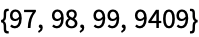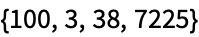# 39 Immediate and Delayed Values

 39 Immediate and Delayed Values
In immediate assignment, the value is computed immediately when the assignment is done, and is never recomputed. In delayed assignment, the computation of the value is delayed, and is done every time the value is requested.
As a simple example, consider the difference between value=RandomColor[] and value:=RandomColor[].
In immediate assignment (=), a random color is immediately generated:
value = RandomColor[ ]

Every time you ask for value, you get the same random color:
value

In delayed assignment (:=), no random color is immediately generated:
value := RandomColor[]
Each time you ask for value, is computed, and a new color is generated:
value

The color will typically be different every time:
value

It’s very common to use := if something isn’t ready yet when you’re defining a value.
You can make a delayed assignment for circles even though n doesn’t yet have a value:
circles := Graphics[Table[Circle[{x, 0}, x/2], {x, n}]]
Give n a value:
n = 6

circles

The idea of delayed assignment is directly analogous to the Delayed construct we discussed for deploying webpages. In delayed assignment we don’t compute a value until we need it. Similarly, when we use CloudPublish with Delayed we don’t compute the content of a webpage until someone asks for it.
There’s a notion of delayed rules too. xrhs computes rhs immediately. But in a delayed rule xrhs (typed : >), rhs is instead recomputed every time it’s requested.
This is an immediate rule, where a specific value for RandomReal[ ] is immediately computed:
x -> RandomReal[]

You can replace four x’s, but they’ll all be the same:
{x, x, x, x} /. x -> RandomReal[]

This is a delayed rule, where the computation of is delayed:
x :> RandomReal[]

is computed separately when each x is replaced, giving four different values:
{x, x, x, x} /. x :> RandomReal[]

39.1Replace x in {x, x+1, x+2, x^2} by the same random integer up to 100. »
Sample expected output:
 Out[]=Answer & check your solution
39.2Replace each x in {x, x+1, x+2, x^2} by a separately chosen random integer up to 100. »
Sample expected output:
 Out[]=Answer & check your solution
Because you don’t want to have to recompute things unless it’s necessary. It’s more efficient to just compute something once, then use the result over and over again.
How does one read := and :> out loud?
:= is usually just “colon equals”, though sometimes “delayed assignment”. :> is usually “colon greater”, though sometimes “delayed rule”.
What happens if I do x=x+1, with x not having a value?
You’ll start an infinite loop that’ll eventually get cut off by the system. x={x} is the same story.
What’s the significance of inputs being labeled In[n]:=, and outputs Out[n]= ?
It indicates that inputs are assigned to In[n] and outputs to Out[n]. The := for input means the assignment is delayed, so that if you ask for In[n] the result will be recomputed.